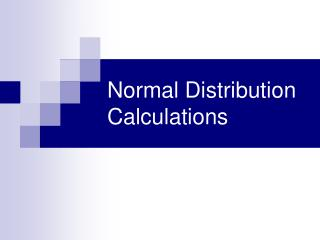DownloadDownload PresentationNormal Distribution Calculations

# Normal Distribution Calculations

Download Presentation## Normal Distribution Calculations

- - - - - - - - - - - - - - - - - - - - - - - - - - - E N D - - - - - - - - - - - - - - - - - - - - - - - - - - -
##### Presentation Transcript

1. Normal Distribution Calculations

2. Using the 4-step Process • State: Express in terms of the observed variable x. • Plan: Draw a picture of the distribution and shade the area of interest. • Do: Perform calculations • Standardize x • Use the table • Conclude: Write your conclusion in the context of the problem.

3. When Tiger Woods hits his driver, the distance the ball travels follows a Normal Distribution with mean 304 yards and standard deviation 8 yards. What percent of Tiger’s drives travel at least 290 yards? • State: • Plan: • Do: • Conclude: Let x = the distance Tiger’s ball travels and it has a distribution N(304, 8). We want P(x  290). About 96% of Tigers drives travel at least 290 yards.

4. Scores for a test have a mean of 100 and standard deviation of 15. Find the probability that a score is below 112.

5. Every month, American households generate an average of 28 pounds of newspaper for garbage or recycling. Assume =2 pounds. If a household is selected at random, find the probability that it generates between 27 and 31 pounds per month.

6. An exclusive college desires to accept only the top 10% of all graduating seniors based on the results of a national placement test. This test has a mean of 500 and a standard deviation of 100. Find the cutoff score for the exam.

7. For a medical study a researcher wishes to select people in the middle 60% of the population based on blood pressure. If the mean systolic blood pressure is 120 and the standard deviation is 8, find the upper and lower reading that would qualify a person to be in the study.

8. In the 2008 Wimbledon tennis tournament, Rafael Nadal averaged 115 miles per hour (mph) on his first serves. Assume that the distribution of his first serve speeds is Normal with a mean of 115 mph and a standard deviation of 6 mph. About what proportion of his first serves would you expect to exceed 120 mph?

9. What percent of Rafael Nadal’s first serves are between 100 and 110 mph? N(115, 6)

10. According to http://www.cdc.gov/growthcharts/, the heights of 3 year old females are approximately Normally distributed with a mean of 94.5 cm and a standard deviation of 4 cm. What is the third quartile of this distribution?

11. Homework • Worksheet (not the book problems listed)